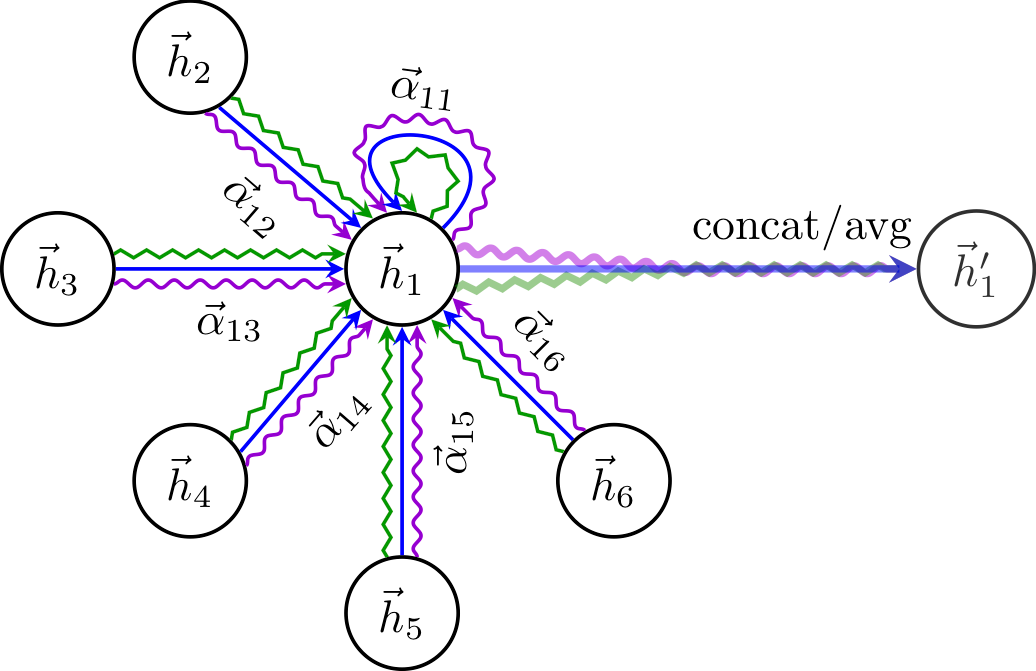# Graph Attention Networks Under the HoodA Step-by-step Guide From Math to NumPy

Graph Neural Networks (GNNs) have emerged as the standard toolbox to learn from graph data. GNNs are able to drive improvements for high-impact problems in different fields, such as content recommendation or drug discovery. Unlike other types of data such as images, learning from graph data requires specific methods. As defined by

Michael Bronstein

:[..] these methods are based on some form of message passing on the graph allowing different nodes to exchange information.

For accomplishing specific tasks on graphs (node classification, link prediction, etc.), a GNN layer computes the node and the edge representations through the so-called recursive neighborhood diffusion (or message passing). According to this principle, each graph node receives and aggregates features from its neighbors in order to represent the local graph structure: different types of GNN layers perform diverse aggregation strategies.

## Graph Neural Networks for Multi-Relational Data

How to extend the simplest formulation of Graph Neural Networks (GNNs) to encode the structure of multi-relational data, such as Knowledge Graphs (KGs) From GCNs to R-GCNs: encoding the structure of Knowledge Graphs with neural architectures (examples in NumPy code)

## NumPy Features - Why we should use Numpy?

Learn numpy features to see why you should use numpy - high performance, multidimensional container, broadcasting functions, working with varied databases

## NumPy Applications - Uses of Numpy

Learn the uses of numpy - Alternate for lists in python, multi dimensional array, mathematical operations. See numpy applications with python libraries.

## How Graph Convolutional Networks (GCN) Work

In this post, we’re gonna take a close look at one of the well-known Graph neural networks named GCN. First, we’ll get the intuition to see how it works, then we’ll go deeper into the maths behind it.

## How to Explain Graph Neural Network — GNNExplainer

Unlike CNN, where we can extract activation of each layer to visualize the decisions of the network, in GNN it is hard to get a meaningful explanation of what features the network has learnt. Here's Step-by-step guide for a GNNExplainer for node and graph explanation implemented in PyTorch Geometric.